## Prefix-Postfix-Expression

 Question 1

The postfix for a string is ABC + -D*, the actual string will be

 A (A-(B+C))*D B ((A-B)+C)*D C ((A+B)-C)*D D (A+(B-C))*D
Data-Structures       Prefix-Postfix-Expression       APPSC-2016-DL-CA
 Question 2
The postfix expression for the infix expression A+B+(C+D)/F+D+E is
 A AB+CD+ *F/D+E* B ABCD+ *F/+ DE* + C A*B+CD/F+DE++ D None of these
Data-Structures       Prefix-Postfix-Expression       TNPSC-2012-Polytechnic-CS
Question 2 Explanation:
The given infix expression is,
(A + B + (C + D))/ (F + D + F)
⇒ Push ‘(’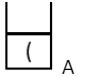⇒ Push ‘+’⇒ Since ‘+’ is Left associative, so ‘+’ in stack will have higher procedure then the current ‘+’, hence pop it,⇒ Push ‘+’⇒ Push ‘(’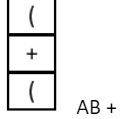⇒ Push ‘+’⇒ Now on looking ‘)’ we will pop all the operators till first ‘(‘,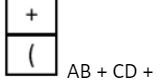⇒ Pop⇒ Push /⇒ Push ‘(’⇒ Push ‘+’⇒ Since + is left associative, so pop ‘+’,⇒ Push up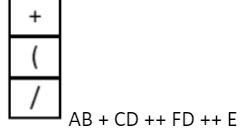⇒ Since ‘(’ came so pop till ‘)’,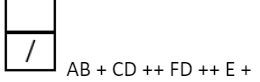⇒ Pop ‘/’Hence, postfix expression is,
AB + CD ++ FD ++ E +/
 Question 3
The value of the postfix expression abc*de+/- where a=20, b=3, c=4, d=1 and e=5 is
 A 134 B -22 C 48 D 18
Data-Structures       Prefix-Postfix-Expression       APPSC-2016-DL-CS
Question 3 Explanation:
Here we will start traversing the expression from left to right and if an operand is encountered then it will be pushed on the top of stack and if an operator is encountered then top two elements will be popped off from the top of stack and current operator is applied on those two operands and then in the end the result of operation will be pushed back on the top of stack. This procedure continues till the whole expression is traversed.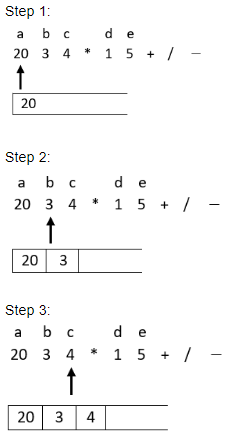Step: 7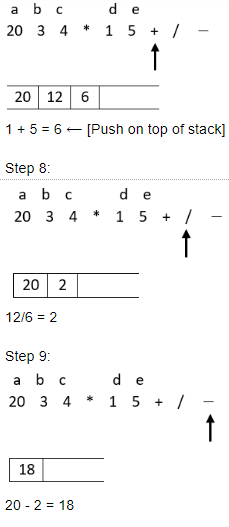Hence option 4 is the correct answer.
 Question 4
The prefix equivalent of the following infix expression is: a / b - c + d * e - a * c
 A - + - / a b c * d e * a c B + - - / a b c * d e * a c C - + / a b c - * d e * a c D - - + / a b c * d e * a c
Data-Structures       Prefix-Postfix-Expression       CIL Part - B
Question 4 Explanation:
a / b - c + d * e - a * c
* Start scanning the expression from RHS. * Push the operators on the top of the stack by ensuring that only high priority operator can be pushed over a low priority operator.
* If a low priority operator is encountered in expression while scanning it, and if top of stack have high priority operator then pop it and after that push low priority operator on top of stack.
* Print each operand just after scanning it.There are 4 questions to complete.

Register Now